# Intermediate Geometry : How to find an angle in an acute / obtuse triangle

## Example Questions

1 3 Next →

### Example Question #21 : How To Find An Angle In An Acute / Obtuse Triangle

Two of the exterior angles of a triangle, taken at different vertices, measure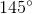and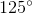. Is the triangle acute, right, or obtuse?

Acute

Obtuse

Right

Right

Explanation:

At a given vertex, an exterior angle and an interior angle of a triangle form a linear pair, making them supplementary - that is, their measures total. The measures of two interior angles can be calculated by subtracting the exterior angle measures from: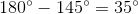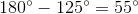The triangle has two interior angles of measures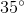and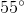. The sum of these measures is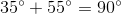, thereby making them complementary. A triangle with two complementary acute angles is a right triangle.

### Example Question #22 : How To Find An Angle In An Acute / Obtuse Triangle

True or false: It is possible for a triangle to have angles of measure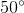, and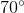.

True

False

True

Explanation:

The sum of the measures of the angles of a triangle is. The sum of the three given angle measures is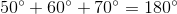.

This makes the triangle possible.

### Example Question #23 : How To Find An Angle In An Acute / Obtuse Triangle

True or false: It is possible for a triangle to have three interior angles, each of whose measures are.

True

False

False

Explanation:

A triangle with three congruent angles is an equiangular - and equilateral - triangle; such an angle must have three angles that measure### Example Question #24 : How To Find An Angle In An Acute / Obtuse Triangle

Given: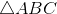with perimeter 40;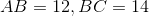True or false: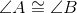True

False

True

Explanation:

The perimeter ofis the sum of the lengths of its sides - that is,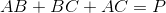The perimeter is 40, so set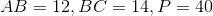, and solve for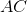: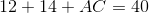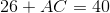Subtract 26 from both sides: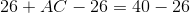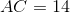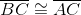, so by the Isosceles Triangle Theorem, their opposite angles are congruent - that is,.

### Example Question #51 : Acute / Obtuse Trianglesis an equilateral triangle;is the midpoint of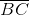; the segmentis constructed.

True or false: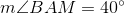.

True

False

False

Explanation:

The referenced triangle is below: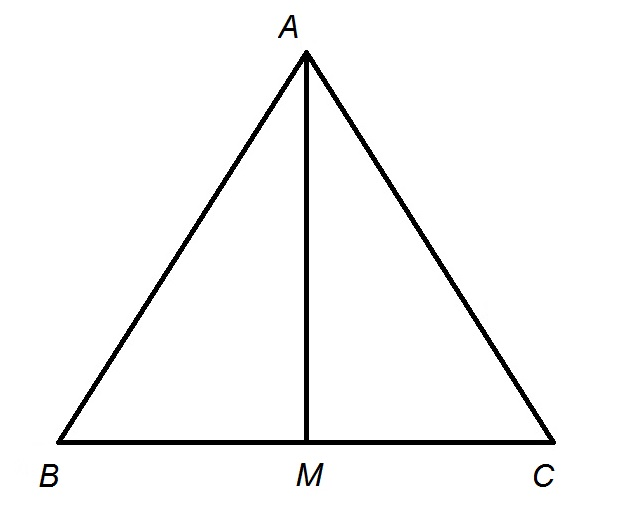In an equilateral triangle, the median from- the segment fromto, the midpoint of the opposite side- is also the bisector of the angle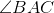, so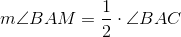Each interior angle of an equilateral triangle, including, measures, so substitute and evaluate: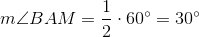.

### Example Question #26 : How To Find An Angle In An Acute / Obtuse Triangleis an equilateral triangle. Locate a pointalongand construct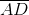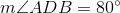Evaluate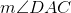.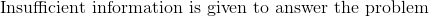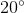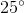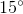Explanation:

The referenced figure is below. Note that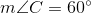, as is the case with all of the interior angles of an equilateral triangle.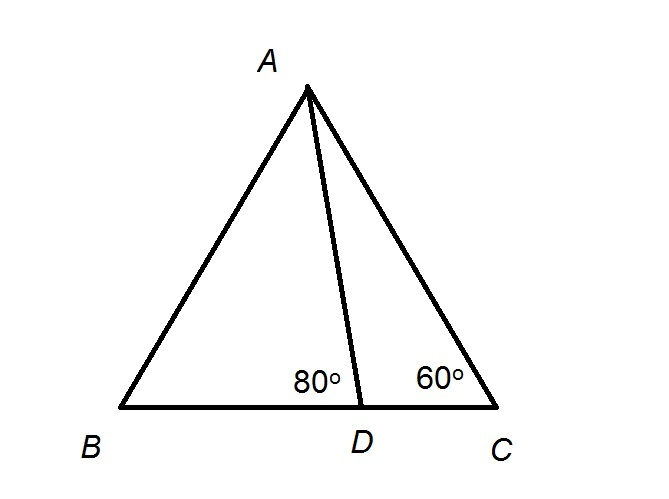The interior angles of an equilateral triangle each measure. An exterior angle of a triangle has as its degree measure the sum of its remote interior angles; specifically,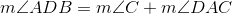Substitute the known angle measures, and solve: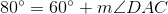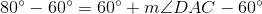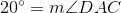1 3 Next →